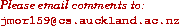Data Structures and Algorithms Finding the Convex Hull

The Convex Hull is the line completely enclosing a set of points in a plane so that there are no concavities in the line. More formally, we can describe it as the smallest convex polygon which encloses a set of points such that each point in the set lies within the polygon or on its perimeter.

### Algorithm

Graham's scan has these steps:
1. Find p0, the point with the minimum y coordinate,
2. Sort all the remaining points in order of their polar angle from p0
3. Initialize a stack, S
4. push(S,p0)
5. push(S,p1)
6. push(S,p2)
7. for i=3 to n do
while (angle formed by topnext(S), top(S) and pi makes a right turn
• pop(S)
push(S,pi
8. return S
This algorithm takes O(n logn) time.

A second algorithm, known as Jarvis' march proceeds as follows:

• Find the 'left-most' (minimum x) and 'right-most' (maximum x) points.
• Divide points into those above and below the line joining these points.
• In the bottom half, starting with the left-most point, add the point with the least angle to the -y axis from the current point until the right-most point is reached,
• Repeat the scan in the upper half.
This algorithm takes O(n h) time, where h is the numer of vertices in the convex hull.

### Animation

Two animations of the convex hull algorithm have been written:
Convex Hull Animation
This animation was written by KyungTae Kim
It is a prototype: volunteers to improve it are welcome!Convex Hull Animation
This animation was written by JiMo Jung
It is a prototype: volunteers to improve it are welcome!### Key terms

convex hull
Line enclosing a set of points in a plane with no concavities.